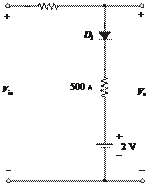### Create an Account

Home / Questions / For the circuit of Figure P914 find the range of Vin for which D1 is forward biased Assu...

# For the circuit of Figure P914 find the range of Vin for which D1 is forward biased Assume an ideal diode

For the circuit of Figure P9.14, find the range of Vin

for which D1 is forward-biased. Assume an ideal diode.+

Vi   = 12 V

-

5 Ù           -   vD

+               10 Ù

+

VB   = 10 V

-

1 kÙFigure P9.7

Jun 18 2020 View more View LessSubscribe To Get Solution